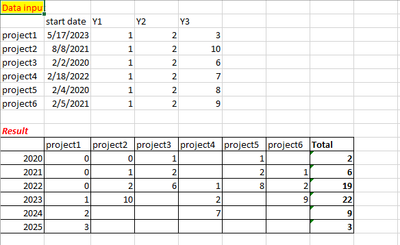cancel
Showing results for
Did you mean:Regular Visitor

## Calculate sum of projects that need investment for 3 years with different start date

Hi all,

I have projects with different start date and projects investment takes for each 3 years and I need a Power BI measure that calculate the investment per year. I have only one table.2 ACCEPTED SOLUTIONSSolution Sage

Hello @Amma17,

Please consider the following solution consisting of 2 steps:

1) You transform your initial table into the new one with the following [DAX] code.2) You add a matrix visual, set it up and enjoy the result.For convenience, here is the [DAX] code in plain text:

``````Table = ADDCOLUMNS (
( GENERATE ( SELECTCOLUMNS ( GENERATESERIES ( MIN ( data[Year] ), MAX ( data[Year] ) + 2 ), "Year", [Value] ),
VALUES ( data[Project] ) ) ),
"Investment",
VAR StartYear = YEAR ( LOOKUPVALUE ( data[Date], data[Project], [Project] ) )
RETURN SWITCH ( TRUE (),
[Year] = StartYear, LOOKUPVALUE ( data[Y1], data[Project], [Project] ),
[Year] = StartYear + 1, LOOKUPVALUE ( data[Y2], data[Project], [Project] ),
[Year] = StartYear + 2, LOOKUPVALUE ( data[Y3], data[Project], [Project] ),
0 )
)      ``````

Best Regards,

AlexanderRegular Visitor

Solve it, I created a new table that contain years and I created a relationship one to one by year, so problem was solved.

3 REPLIES 3Regular Visitor

I have another question:
How can I create another table with Investment, but split between investment proposal ( created with :

( GENERATE ( SELECTCOLUMNS ( GENERATESERIES ( MIN ( P_N[start date].[Year] ), MAX ( P_N[start date].[Year] ) + 2 ), "Year", [Value] ),
VALUES ( P_N[Column1] ) ) ),
"Investment propose",
VAR StartYear = YEAR ( LOOKUPVALUE ( P_N[start date], P_N[Column1], [Column1] ) )
RETURN SWITCH ( TRUE (),
[Year] = StartYear, LOOKUPVALUE ( P_N[Y1] ,P_N[Column1], [Column1] ),
[Year] = StartYear + 1, LOOKUPVALUE ( P_N[Y1] ,P_N[Column1], [Column1] ),
[Year] = StartYear + 2, LOOKUPVALUE (P_N[Y1] ,P_N[Column1], [Column1] ),
0 )
) and next to him investment realized, follow the same rule as investment proposal.
2.How can I fix investment proposal in the picture below to show me the real investment not the accumulated investment
in each row(third table).Regular Visitor

Solve it, I created a new table that contain years and I created a relationship one to one by year, so problem was solved.Solution Sage

Hello @Amma17,

Please consider the following solution consisting of 2 steps:

1) You transform your initial table into the new one with the following [DAX] code.2) You add a matrix visual, set it up and enjoy the result.For convenience, here is the [DAX] code in plain text:

``````Table = ADDCOLUMNS (
( GENERATE ( SELECTCOLUMNS ( GENERATESERIES ( MIN ( data[Year] ), MAX ( data[Year] ) + 2 ), "Year", [Value] ),
VALUES ( data[Project] ) ) ),
"Investment",
VAR StartYear = YEAR ( LOOKUPVALUE ( data[Date], data[Project], [Project] ) )
RETURN SWITCH ( TRUE (),
[Year] = StartYear, LOOKUPVALUE ( data[Y1], data[Project], [Project] ),
[Year] = StartYear + 1, LOOKUPVALUE ( data[Y2], data[Project], [Project] ),
[Year] = StartYear + 2, LOOKUPVALUE ( data[Y3], data[Project], [Project] ),
0 )
)      ``````

Best Regards,

AlexanderAnnouncements#### Exclusive opportunity for Women!

Join us for a free, hands-on Microsoft workshop led by women trainers for women where you will learn how to build a Dashboard in a Day!#### Power Platform Conference-Power BI and Fabric Sessions

Join us Oct 1 - 6 in Las Vegas for the Microsoft Power Platform Conference.Top Solution Authors
Top Kudoed Authors
Users online (2,198)